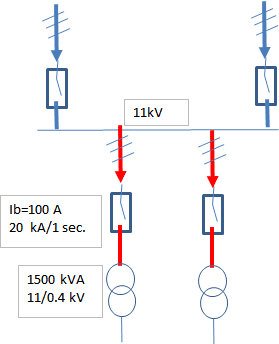# How to calculate the cable size between QRM and transformer

cable size calculation
between QRM and 11kv to 400v transformer (1500 kva)

anorlunda
Staff Emeritus
jim hardy
Gold Member
Dearly Missed
What's a QRM?

Is your cable copper or aluminum, is it run in air or in conduit, and if buried what is thermal conductivity of earth covering it?
What is temperature rating of your insulation ?

That's why we have electrical codes, so that one avoids 'beginner's mistakes' .

•dlgoff
QRM has many meanings, I agree with you Jim. Anyway, in my opinion, the o.p. is
referring to Quadrilateral Ring Main Unit. RMU (Ring Main Unit) is of two types. TRM (Triangular Ring Main Unit) & QRM (Quadrilateral Ring Main Unit). TRM is (2 Feeder+ 1 Transformer Feeder) and QRM is (2 Feeder + 2 Transformer Feeder). RMU is mainly used for redundancy of feeders.
So, a sketch it could explain more.The short-circuit current will state the cable cross-section area. If you know the circuit breaker short-circuit rated current then you may use the NEC Table 240.92(B) Tap Conductor Short-Circuit Current Ratings.[for copper conductor]:
A=I/SQRT(0.0297*LOG10((T2+234)/(T1+234))/t) where:
I = short-circuit current in amperes
A = conductor area in circular mils
t = time of short circuit in seconds (for times less than or equal to 10 seconds)
T1 = initial conductor temperature in degrees Celsius.
T2 = final conductor temperature in degrees Celsius.
T1=75oC usually T2=250oC for XLPE or EPR conductor insulation.
Let's say I=20000 A T2=250oC t=1 sec T1=75oC then A= A=26300 cmls [=135 mm^2].

#### Attachments

•Fisherman199 and jim hardy
jim hardy
•